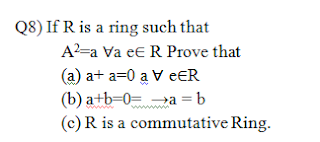## Discrete Structures Question Paper of 3rd Semester CSE74 Download Previous Years Question Paper 16

•Thursday, September 08, 2016
••,
•No commentsRoll No……..
Total No. of Questins:9]
B.Tech. (Sem. – 3rd )
DISCRETE STRUCTURES
SUBJECT CODE : CS - 203
Paper ID : [A0452]
Time : 03 Hours
Instruction to Candidates:
1) Section - A is Compulsory.
2) Attempt any Four questions from Section - B.
3) Attempt any Two questions from Section - C.

Section - A
QI) Define following terms with examples.
a) Euler graph.
b) Poset.
c) Lattice.
d) Ring.
e) Group.
0 Quotient ring.
g) Integral domain.
h) Semi group.
i) Reflexive relation.
j) Function.
Section - B

Q2) For A: { I ,2,{ 1,3}, ɸ}, determine the following sets.

(a) A-{1} (b) A- ɸ (c) A-{ ɸ } (d) A-{1,2,).

Q3) Write all possible relations from

A: {0} to B: {1,2}

Q4) Give an explicit formula for a function from the set of integers to the set of positive integers i.e.

(a) One to one but not onto.
(b) Onto but not one to one.
(c) One to one and onto.
(d) Neither one to one nor onto.

Q5) In how many ways can 5 Gentle man and 5 ladies be seated round a table so that no two ladies are together.

Q6) Show that following graphs are planar.

Section - C

Q7) (a) Suppose 8 people enter a Badminton tournament. Use a rooted tree model of the tournament                determine how many games must he played to determine a champion if a player is eliminated              after one loss.

(b) Prove that the set G: {1, 2,3,4,5,6} is a finite alielian Groups of order 6 with respect to                 multiplication modulo 7.

Q9) (a) Simplify using Boolean postulates and theorems

a +ab + abc + abcd + a + ab + abc + abcd

(b) Discuss various applications of Boolean Algebra'